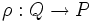# P-quotient-pullbackable automorphism

This article defines an automorphism property, viz a property of group automorphisms. Hence, it also defines a function property (property of functions from a group to itself)
View other automorphism properties OR View other function properties

## Definition

Let$P$ be a group of prime power order, i.e., a finite$p$-group for some prime$p$. An automorphism$\sigma$ of$P$ is termed a p-quotient-pullbackable automorphism if, given any finite$p$-group$Q$ and a surjective homomorphism$\rho:Q \to P$, there exists an automorphism$\sigma'$ of$Q$ that is a pullback of$\sigma$; in other words,$\rho \circ \sigma' = \sigma \circ \rho$.

## Relation with other properties

### Stronger properties

• Inner automorphism of a finite$p$-group.

### Weaker properties

• Cofactorial automorphism: Any$p$-quotient-pullbackable automorphism of a finite$p$-group is itself a$p$-automorphism. For full proof, refer: p-quotient-pullbackable automorphism implies p-automorphism